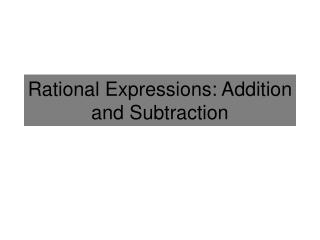DownloadDownload Presentation# Rational Expressions: Addition and Subtraction

Télécharger la présentation## Rational Expressions: Addition and Subtraction

- - - - - - - - - - - - - - - - - - - - - - - - - - - E N D - - - - - - - - - - - - - - - - - - - - - - - - - - -
##### Presentation Transcript

1. Rational Expressions: Addition and Subtraction

2. Adding and Subtracting Fractions Simplify the following expressions: If you can, find the Least Common Denominator Find a Common Denominator Subtract the Numerators Add the Numerators Remember to Simplify if Possible!

3. Add/Subtract Rational Expressions State the values that x can not equal, then simplify: Same denominator! Half the work is done! CAREFUL with subtraction. Don’t forget to subtract each term. Excluded values of x: Find when the denominator(s) is 0: Combine Like Terms 5 and -3. Cancel Make sure it can’t be simplified more These Make the ORIGINAL denominator equal 0. We assume that x can never be these values.

4. Add/Subtract Rational Expressions Simplify: In order to add the fractions, find a Common Denominator (2x2)(x)=2x3 is a common denominator, but if possible find a LEAST common denominator. Since the denominators are equal, add the numerators

5. Add/Subtract Rational Expressions Simplify: The easiest way to find a common denominator, is to multiply by the other given denominators. In order to subtract, find a common denominator Subtract since the denominators are equal, subtract. But be CAREFUL with subtraction. Make sure to distribute the negative if necessary. Distribute numerators but leave the denominators factored Combine like Terms

6. Add/Subtract Rational Expressions Simplify, assuming x≠0,1/3: If possible, factor to find a Smaller Common Denominator If possible, simplify before subtracting the fractions. Since the denominators are equal, subtract the numerators Combine like Terms

7. Add/Subtract Rational Expressions Simplify, assuming x≠-4,4: If possible, factor to find a Smaller Common Denominator The easiest way to find a common denominator, is to multiply by the factors of the other given denominators it is missing. Since the denominators are equal, add the numerators Factor to make sure it is simplified.